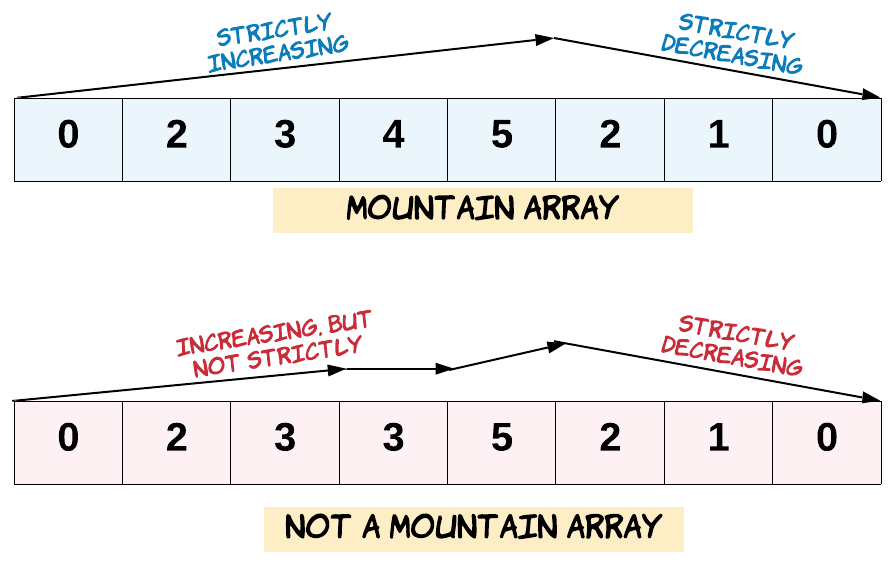# PHP 求解有效的山脉数组

## PHP 求解有效的山脉数组

author: he xiaodong date: 2020-11-04
##### 有效的山脉数组• A.length >= 3
• 在 0 < i < A.length - 1 条件下，存在 i 使得：
• A < A < … A[i-1] < A[i]
• A[i] > A[i+1] > … > A[A.length - 1]

``````输入：[2,1]

``````

``````输入：[3,5,5]

``````

``````输入：[0,3,2,1]

``````

• 0 <= A.length <= 10000
• 0 <= A[i] <= 10000

##### 解题思路

``````class Solution {

/**
* @param Integer[] \$A
* @return Boolean
*/
function validMountainArray(\$A) {
if (count(\$A) < 3) return false;
\$left = 0;
\$right = count(\$A) - 1;

// 注意防止越界
while (\$left < count(\$A) - 1 && \$A[\$left] < \$A[\$left + 1]) \$left++;

// 注意防止越界
while (\$right > 0 && \$A[\$right] < \$A[\$right - 1]) \$right--;

// 如果left或者right都在起始位置，说明不是山峰
if (\$left == \$right && \$left != 0 && \$right != count(\$A) - 1) return true;
return false;
}
}
``````